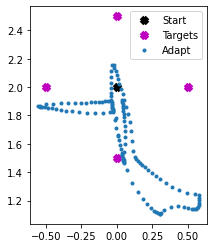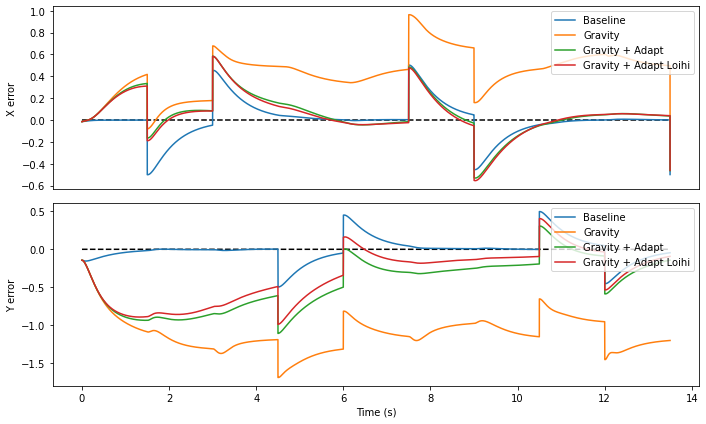In this example, we will use the PES learning rule to learn the effects of gravity, applied to a 2-joint arm.

This example requires the abr_control library. To install it,

git clone https://github.com/abr/abr_control.git
pip install -e abr_control


Note that while this example uses a simualted 2-joint arm, the underlying network structure is identical to the network previously used to control a physical robot arm.

:

%matplotlib inline
from abr_control.arms import twojoint as arm
from abr_control.controllers import OSC
import matplotlib.pyplot as plt
import nengo
import numpy as np
import nengo_loihi

nengo_loihi.set_defaults()


## Creating the arm simulation and reaching framework¶

The first thing to do is create the arm simulation to simulate the dynamics of a two link arm, and an operational space controller to calculate what torques to apply to the joints to move the hand in a straight line to the targets.

:

# set the initial position of the arm
robot_config = arm.Config(use_cython=True)
arm_sim = arm.ArmSim(robot_config=robot_config, dt=1e-3, q_init=[0.95, 2.0])

# create an operational space controller
ctrlr = OSC(robot_config, kp=10, kv=7, vmax=[10, 10])

Robot has fewer DOF (2) than the specified number of space dimensions to control (3), Poor performance may result.



Next we create a set of targets for the arm to reach to. In this task the hand will start at a center location, then reach out and back to 4 targets around a circle.

:

# create a set of targets to reach to
n_reaches = 4
seconds_per_target = 1.5

distance = 0.5
center = [0, 2.0]
end_points = [
[distance * np.cos(theta) + center, distance * np.sin(theta) + center]
for theta in np.linspace(0, 2 * np.pi, n_reaches + 1)
][:-1]
targets = np.vstack([[center, ep] for ep in end_points])

plt.plot(center, center, "bx", mew=5, label="Start")
for i, end_point in enumerate(end_points):
plt.plot(end_point, end_point, "x", mew=5, label=f"Target {i + 1}")
plt.gca().set_aspect("equal")
plt.legend()

:

<matplotlib.legend.Legend at 0x7f3ede3e80a0>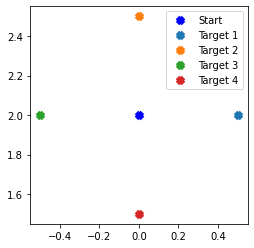:

def build_baseline_model():
with nengo.Network(label="Nonlinear adaptive control") as model:

# Create node that specifies target
def gen_target(t):
# Advance to the next target in the list every 2 seconds
return targets[int(t / seconds_per_target) % len(targets)]

target_node = nengo.Node(output=gen_target, size_out=2)

# Create node that calculates the OSC signal
model.osc_node = nengo.Node(
output=lambda t, x: ctrlr.generate(
q=x[:2], dq=x[2:4], target=np.hstack([x[4:6], np.zeros(4)])
),
size_in=6,
size_out=2,
)

# Create node that runs the arm simulation and gets feedback
def arm_func(t, x):
u = x[:2]  # the OSC signal
u += x[2:4] * 10  # add in the adaptive control signal
arm_sim.send_forces(u)  # step the arm simulation forward
# return arm joint angles, joint velocities, and hand (x,y)
return np.hstack([arm_sim.q, arm_sim.dq, arm_sim.x])

model.arm_node = nengo.Node(output=arm_func, size_in=4)

# hook up the OSC controller and arm simulation
nengo.Connection(model.osc_node, model.arm_node[:2])
nengo.Connection(model.arm_node[:4], model.osc_node[:4])
# send in current target to the controller
nengo.Connection(target_node, model.osc_node[4:6])

model.probe_target = nengo.Probe(target_node)  # track targets
model.probe_hand = nengo.Probe(model.arm_node[4:6])  # track hand (x,y)
model.probe_q = nengo.Probe(model.arm_node[:2])  # track joint angles

return model

baseline_model = build_baseline_model()


## Running the network in Nengo¶

We can now run the basic framework, where the operational space controller will drive the hand to the 4 targets around the circle.

:

# each reach is seconds_per_target seconds out + the same back in
runtime = seconds_per_target * (2 * n_reaches + 1)

arm_sim.reset()
with nengo.Simulator(baseline_model, progress_bar=False) as sim:
sim.run(runtime)
baseline_t = sim.trange()
baseline_data = sim.data


The error is calculated as the difference between the hand (x,y) position and the target. Whenever the target changes, the error will jump up, and then quickly decrease as the hand approaches the target.

:

def calculate_error(model, data):
return data[model.probe_hand] - data[model.probe_target]

baseline_error = calculate_error(baseline_model, baseline_data)

def plot_data(t_set, data_set, label_set):
plt.figure(figsize=(10, 6))
plt.title("Distance to target")

ax1 = plt.subplot(2, 1, 1)
ax1.plot(t_set, np.zeros_like(t_set), "k--")
plt.xticks([])
plt.ylabel("X error")

ax2 = plt.subplot(2, 1, 2)
ax2.plot(t_set, np.zeros_like(t_set), "k--")
plt.ylabel("Y error")
plt.xlabel("Time (s)")

plt.tight_layout()

for t, data, label in zip(t_set, data_set, label_set):
ax1.plot(t, data[:, 0], label=label)
ax2.plot(t, data[:, 1], label=label)

ax1.legend(loc=1)
ax2.legend(loc=1)

def plot_xy(t_set, data_set, label_set):
tspace = 0.1
ttol = 1e-5

plt.figure()
ax = plt.subplot(111)
ax.plot(center, center, "kx", mew=5, label="Start")
ax.plot(
[p for p in end_points],
[p for p in end_points],
"mx",
mew=5,
label="Targets",
)
for t, data, label in zip(t_set, data_set, label_set):
tmask = (t + ttol) % tspace < 2 * ttol
ax.legend(loc=1)
ax.set_aspect("equal")

plot_xy([baseline_t], [baseline_data[baseline_model.probe_hand]], ["Baseline"])
plot_data([baseline_t], [baseline_error], ["Baseline"])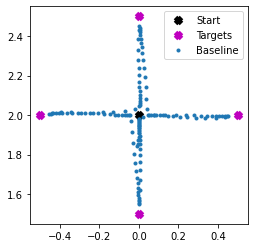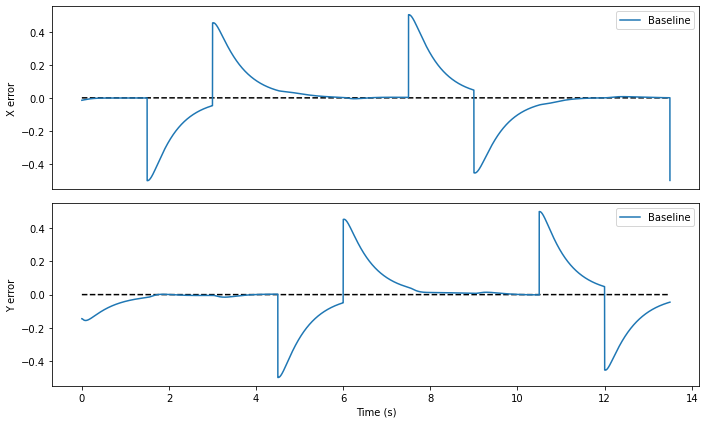Now we add gravity along the y-axis to the 2-link arm model, which it is not expecting. As a result, the error will be much greater as it tries to reach to the various targets relative to baseline performance. Note that although gravity is only applied along the y-axis, because the arm joints are not all oriented vertically, the effect of gravity on the arm segments also pulls the hand along the x-axis.

:

def add_gravity(model):
with model:
# calculate and add in gravity along y axis
gravity = np.array([0, -9.8, 0, 0, 0, 0])

def gravity_func(t, q):
return g

gravity_node = nengo.Node(gravity_func, size_in=2, size_out=2)
# connect perturbation to arm
nengo.Connection(model.arm_node[:2], gravity_node)
nengo.Connection(gravity_node, model.arm_node[:2])

return model


:

arm_sim.reset()
with nengo.Simulator(gravity_model, progress_bar=False) as sim:
sim.run(runtime)
gravity_t = sim.trange()
gravity_data = sim.data

:

gravity_error = calculate_error(gravity_model, gravity_data)
plot_xy([gravity_t], [gravity_data[gravity_model.probe_hand]], ["Gravity"])
plot_data(
[baseline_t, gravity_t], [baseline_error, gravity_error], ["Baseline", "Gravity"]
)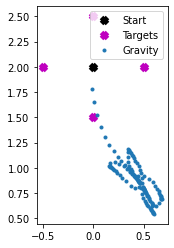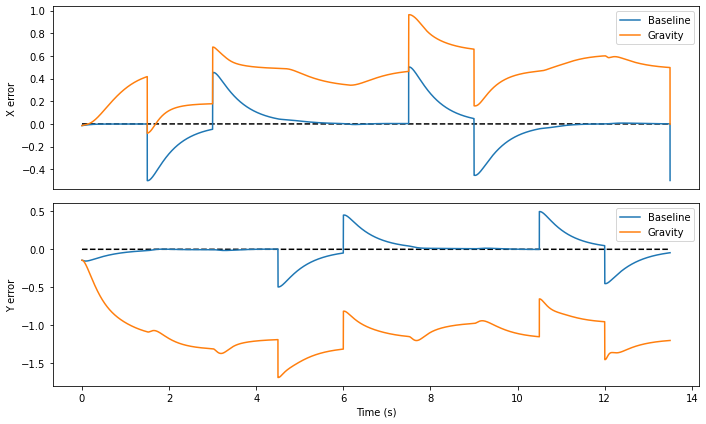As expected, the error is much worse, especially along the Y axis (take note of the scale of the axes), because gravity is affecting the system and the operational space controller is not accounting for it.

Now we add in an ensemble of neurons to perform context sensitive error integration, as presented in DeWolf, Stewart, Slotine, and Eliasmith, 2015. We want the context for adaptation to be the joint angles of the arm. For optimal performance, the input to the ensemble should be in the range -1 to 1, so we need to look at the expected range of joint angles and transform the input signal accordingly.

:

for ii in range(2):
plt.subplot(2, 1, ii + 1)
plt.plot(
sim.trange(), baseline_data[baseline_model.probe_q][:, ii], label="Baseline"
)
plt.plot(sim.trange(), gravity_data[gravity_model.probe_q][:, ii], label="Gravity")
plt.ylabel("Joint %i" % ii)
plt.legend()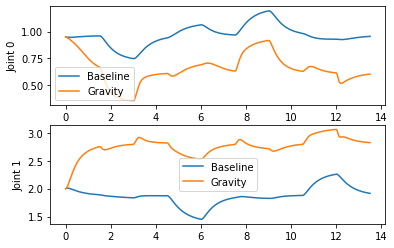Looking at this data, we can estimate the mean of Joint 0 across the two runs to be around .75, with a range of .75 to either side of the mean. For Joint 1 it’s a bit more difficult; we’re going to err to the Baseline values with the hope the adaptive controller helps keep us in that range. So we’ll choose a mean of 2 and range of 1 to either side of the mean.

:

def add_adaptation(model, learning_rate=1e-5):
with model:
# create ensemble to adapt to unmodeled dynamics

means = np.array([0.75, 2.25])
scaling = np.array([0.75, 1.25])
scale_node = nengo.Node(
output=lambda t, x: (x - means) / scaling, size_in=2, size_out=2
)
# to send target info to ensemble
nengo.Connection(model.arm_node[:2], scale_node)

# create the learning connection from adapt to the arm simulation
learn_conn = nengo.Connection(
model.arm_node[2:4],
function=lambda x: np.zeros(2),
learning_rule_type=nengo.PES(learning_rate),
synapse=0.05,
)
# connect up the osc signal as the training signal
nengo.Connection(model.osc_node, learn_conn.learning_rule, transform=-1)
return model


:

arm_sim.reset()
sim.run(runtime)

:

adapt_error = calculate_error(adapt_model, adapt_data)
plot_data(
)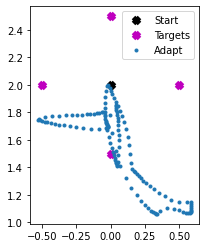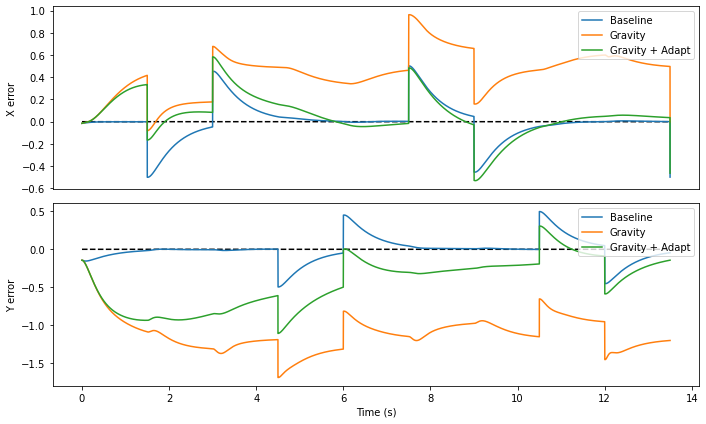And we can see that even in only one pass through the target set the adaptive ensemble has already significantly reduced the error. To see further reductions, change the run time of the simulation to be longer so that the arm does multiple passes of the target set (by changing sim.run(runtime) to sim.run(runtime*2)).

## Running the network with NengoLoihi¶

Loihi has limits on the magnitude of the error signal, so we need to adjust the pes_error_scale parameter to avoid too much overflow.

:

arm_sim.reset()

loihi_model = nengo_loihi.builder.Model()
loihi_model.pes_error_scale = 10

sim.run(runtime)

/home/tbekolay/Code/nengo-loihi/nengo_loihi/builder/discretize.py:180: UserWarning: Received PES error greater than chip max (1.27e+01). Consider changing Model.pes_error_scale.
warnings.warn(
/home/tbekolay/Code/nengo-loihi/nengo_loihi/builder/discretize.py:190: UserWarning: Received PES error less than chip min (-1.27e+01). Consider changing Model.pes_error_scale.
warnings.warn(

:

adapt_loihi_error = calculate_error(adapt_model, adapt_loihi_data)